## 11-2 PCA (ä¸»č??†é??†ć?)

chinese version

First of all, to verify the functionity of PDA, we can display PCA-generated basis for an ovally-distributed dataset, as shown next.

Example 1: pca01.mclear j dataNum = 1000; data = randn(1,dataNum)+j*randn(1,dataNum)/3; data = data*exp(j*pi/6); % Rotate 30 degree data = data-mean(data); % Mean subtraction plot(real(data), imag(data), '.'); axis image; DS.input=[real(data); imag(data)]; [DS2, v, eigValue] = pca(DS); v1 = v(:, 1); v2 = v(:, 2); arrow = [-1 0 nan -0.1 0 -0.1]+1+j*[0 0 nan 0.1 0 -0.1]; arrow1 = 2*arrow*(v1(1)+j*v1(2))*eigValue(1)/dataNum; arrow2 = 2*arrow*(v2(1)+j*v2(2))*eigValue(2)/dataNum; line(real(arrow1), imag(arrow1), 'color', 'r', 'linewidth', 4); line(real(arrow2), imag(arrow2), 'color', 'k', 'linewidth', 4); title('Axes for PCA');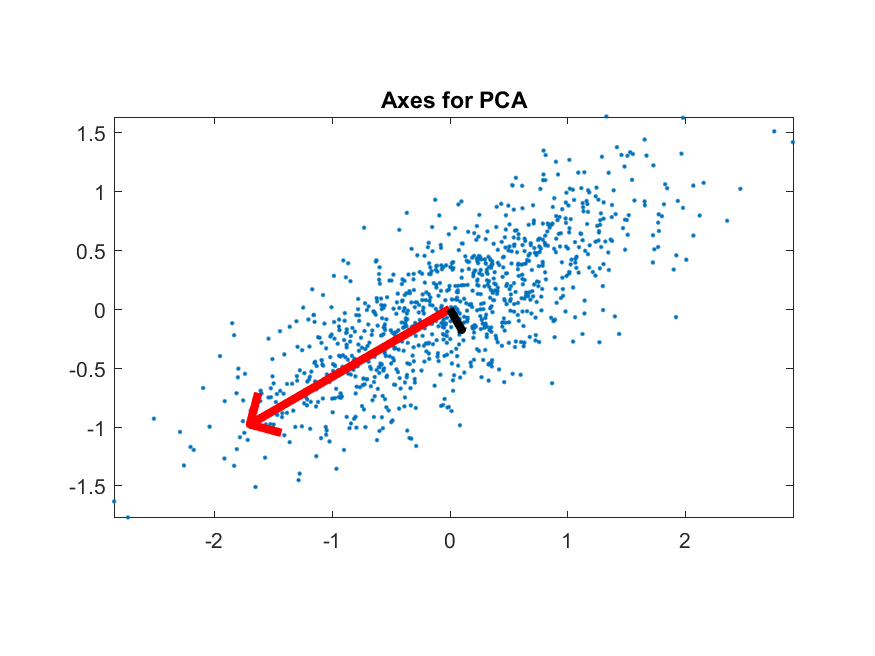It is obvious that the principal component (first direction for the projection basis) is along the direction where the data dispersion after projection is maximized.

In the next example, we perform PCA on the 150 entries of IRIS dataset:

Example 2: pcaIris01.mDS=prData('iris'); DS2=pca(DS); DS3=DS2; DS3.input=DS3.input(1:2, :); % Keep the first two dimenions subplot(2,1,1); dsScatterPlot(DS3); axis image xlabel('Input 1'); ylabel('Input 2'); title('IRIS projected onto the first two dim of PCA'); DS3=DS2; DS3.input=DS3.input(end-1:end, :); % Keep the last two dimenions subplot(2,1,2); dsScatterPlot(DS3); axis image xlabel('Input 3'); ylabel('Input 4'); title('IRIS projected onto the last two dim of PCA');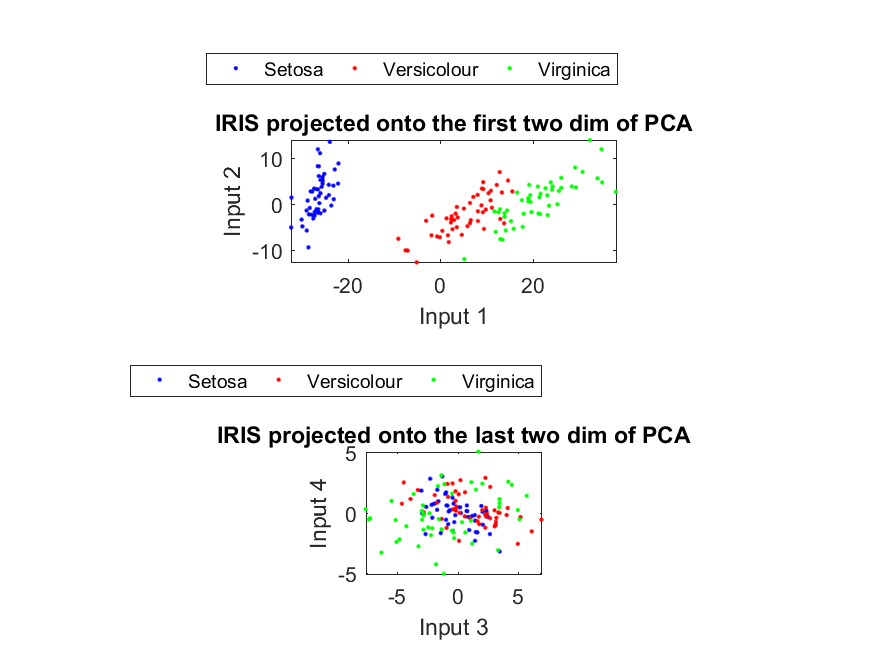The first plot demonstrates the projection of the dataset along the first and second principal components, while the second plot displays the same dataset projection along the third and fourth principal components. Again, it is obvious that the first plot has a wider dispersion than the second plot. (Note that the second plot has a much smaller range than the first plot, indicating the variance after projection is also much smaller.)

For WINE dataset, we can perform similiar computation, as follows:

Example 3: pcaWine01.mDS=prData('wine'); DS2=pca(DS); DS3=DS2; DS3.input=DS3.input(1:2, :); % Keep the first two dimensions subplot(2,1,1); dsScatterPlot(DS3); axis image xlabel('Input 1'); ylabel('Input 2'); title('WINE projected onto the first two dim of PCA'); DS3=DS2; DS3.input=DS3.input(end-1:end, :); % Keep the last two dimensions subplot(2,1,2); dsScatterPlot(DS3); axis image xlabel('Input 12'); ylabel('Input 13'); title('WINE projected onto the last two dim of PCA');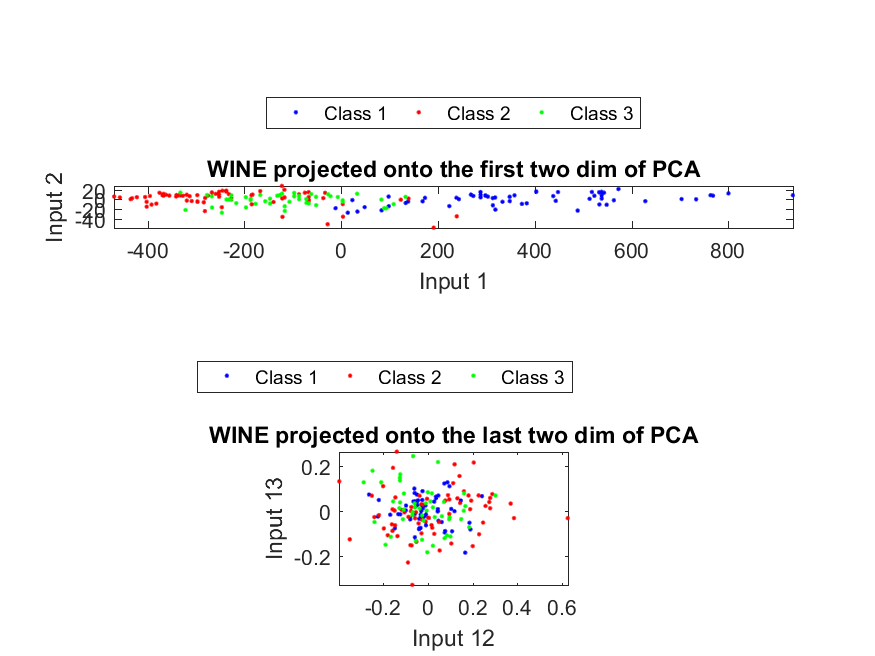Again, the variance of the first plot is much larger than the second plot.

Hint
Though both IRIS and WINE datasets have class labels, we did not use this information along our calculation of PCA.

The goal of PCA is to have the maximum variance after projection, where the class labels, if exist, are not considered in the projection. As a result, PCA is not really optimized for datasets of classification problems. However, since "maximum variance after projection" and "maximum separation between classes after projection" have some characteristics in common, sometimes we use PCA for classification problems too. For instance, in the case of face recognition, the dimension of each face image is so large, and we need to apply PCA for dimension reduction and thus better accuracy.

In the next example, we test the effects of PCA dimension reduction on the classification accuracy of IRIS dataset via KNNC and leave-one-out:

Example 4: pcaIrisDim01.mDS=prData('iris'); [featureNum, dataNum] = size(DS.input); [recogRate, computed] = knncLoo(DS); fprintf('All data ===> LOO recog. rate = %d/%d = %g%%\n', sum(DS.output==computed), dataNum, 100*recogRate); DS2 = pca(DS); recogRate=[]; for i = 1:featureNum DS3=DS2; DS3.input=DS3.input(1:i, :); [recogRate(i), computed] = knncLoo(DS3); fprintf('PCA dim = %d ===> LOO recog. rate = %d/%d = %g%%\n', i, sum(DS3.output==computed), dataNum, 100*recogRate(i)); end plot(1:featureNum, 100*recogRate, 'o-'); grid on xlabel('No. of projected features based on PCA'); ylabel('LOO recognition rates using KNNC (%)');All data ===> LOO recog. rate = 144/150 = 96% PCA dim = 1 ===> LOO recog. rate = 133/150 = 88.6667% PCA dim = 2 ===> LOO recog. rate = 144/150 = 96% PCA dim = 3 ===> LOO recog. rate = 144/150 = 96% PCA dim = 4 ===> LOO recog. rate = 144/150 = 96%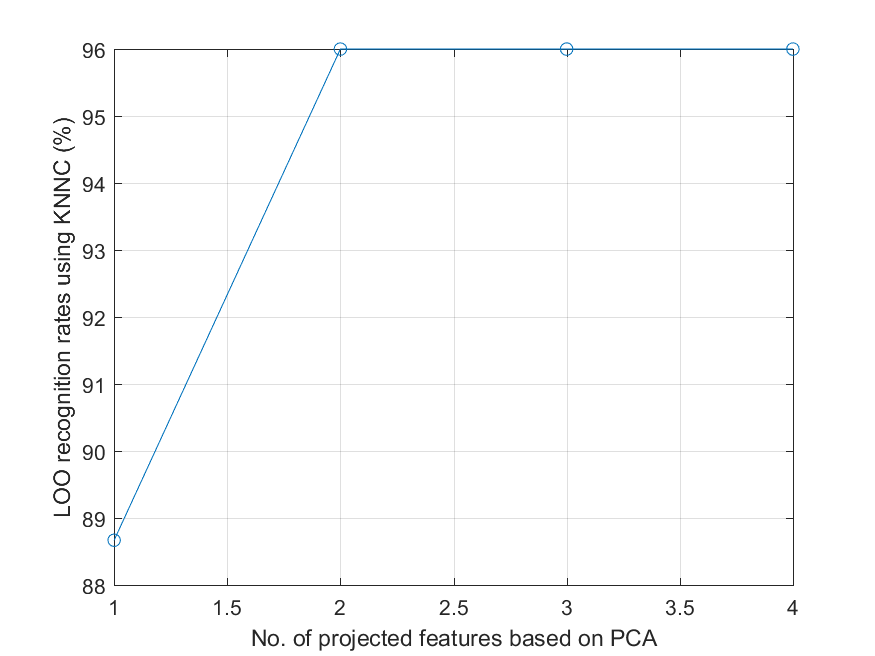If we apply the same procedure for WINE dataset, the result is shown next:

Example 5: pcaWineDim01.mDS=prData('wine'); [featureNum, dataNum] = size(DS.input); [recogRate, computed] = knncLoo(DS); fprintf('All data ===> LOO recog. rate = %d/%d = %g%%\n', sum(DS.output==computed), dataNum, 100*recogRate); DS2 = pca(DS); recogRate=[]; for i = 1:featureNum DS3=DS2; DS3.input=DS3.input(1:i, :); [recogRate(i), computed] = knncLoo(DS3); fprintf('PCA dim = %d ===> LOO recog. rate = %d/%d = %g%%\n', i, sum(DS3.output==computed), dataNum, 100*recogRate(i)); end plot(1:featureNum, 100*recogRate, 'o-'); grid on xlabel('No. of projected features based on PCA'); ylabel('LOO recognition rates using KNNC (%)');All data ===> LOO recog. rate = 137/178 = 76.9663% PCA dim = 1 ===> LOO recog. rate = 126/178 = 70.7865% PCA dim = 2 ===> LOO recog. rate = 128/178 = 71.9101% PCA dim = 3 ===> LOO recog. rate = 130/178 = 73.0337% PCA dim = 4 ===> LOO recog. rate = 135/178 = 75.8427% PCA dim = 5 ===> LOO recog. rate = 136/178 = 76.4045% PCA dim = 6 ===> LOO recog. rate = 137/178 = 76.9663% PCA dim = 7 ===> LOO recog. rate = 137/178 = 76.9663% PCA dim = 8 ===> LOO recog. rate = 137/178 = 76.9663% PCA dim = 9 ===> LOO recog. rate = 137/178 = 76.9663% PCA dim = 10 ===> LOO recog. rate = 137/178 = 76.9663% PCA dim = 11 ===> LOO recog. rate = 137/178 = 76.9663% PCA dim = 12 ===> LOO recog. rate = 137/178 = 76.9663% PCA dim = 13 ===> LOO recog. rate = 137/178 = 76.9663%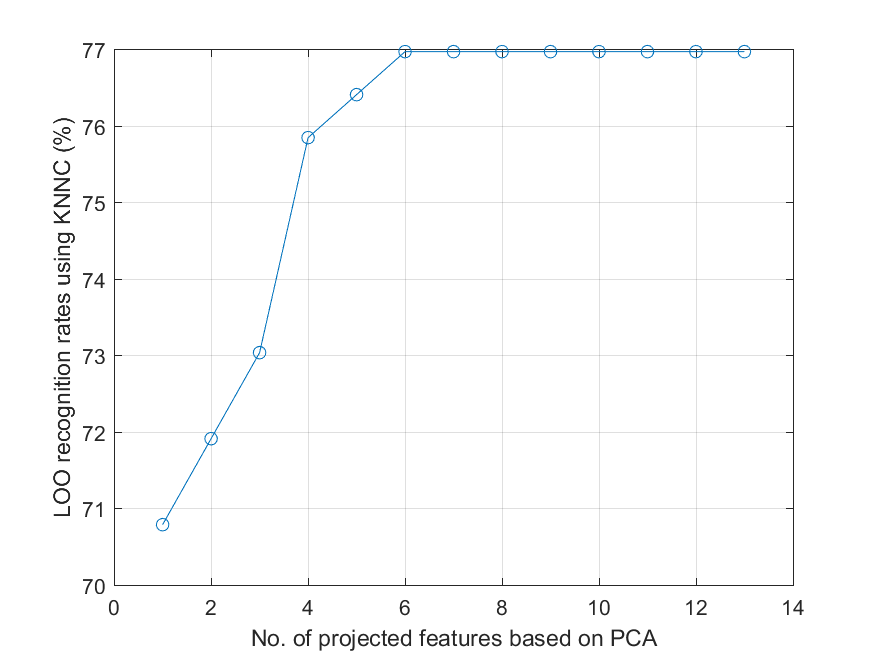From the above two examples, it seems the more features (after PCA projection) we have, the better accuracy we obtain. In other words, straightforward application of PCA cannot select the most effective features for classification. This is reasonable since the class labels are not used for determining the basis for projection of PCA. (Please compare the results with those obtained from LDA in the next section.)

We can convert the above two examples into a function pcaKnncLoo.m for testing the effects of PCA dimension reduction. By using the function, we can try data normalization to see how it can improve the accuracy, as follows:

Example 6: pcaWineDim02.mDS=prData('wine'); recogRate1=pcaPerfViaKnncLoo(DS); DS2=DS; DS2.input=inputNormalize(DS2.input); % data normalization recogRate2=pcaPerfViaKnncLoo(DS2); [featureNum, dataNum] = size(DS.input); plot(1:featureNum, 100*recogRate1, 'o-', 1:featureNum, 100*recogRate2, '^-'); grid on legend('Raw data', 'Normalized data'); xlabel('No. of projected features based on LDA'); ylabel('LOO recognition rates using KNNC (%)');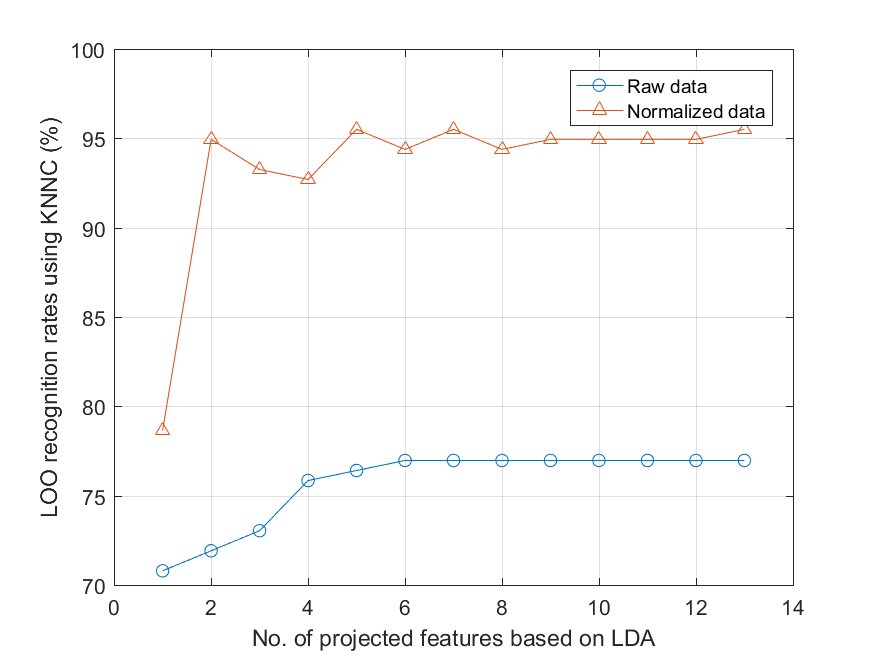From the example, it is obvious that data normalization does improve the accuracy significantly for this dataset. (But the overall accuracy is still not as good as LDA, as explained in the next section.)

Hint
Data normalization does not always guarantee improvement in accuracy. It depends on the dataset as well as the classifier used for performance evaluation.

Hint
In fact, the leave-one-out evaluation used here is a little bit biased toward the optimistic side since we have use all the dataset for PCA projection. In other words, PCA has seen all the dataset already.